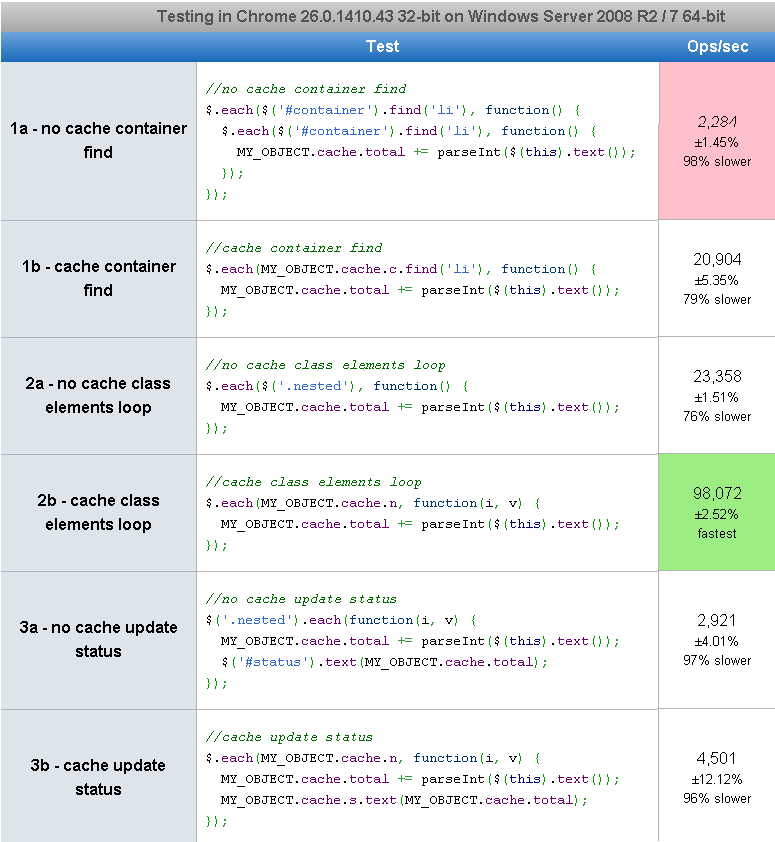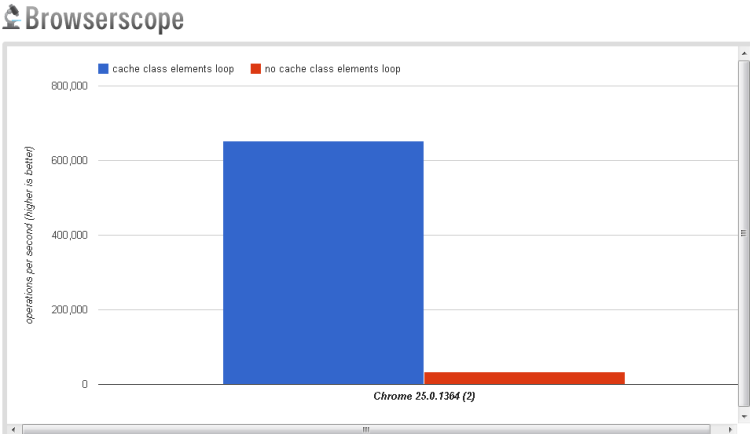# jQuery: The Performance of DOM caching

I decided to test the performance of DOM caching in a basic JavaScript namespacing pattern which I use quite a lot when coding on a day to day basis. Update 10/04/2013: I’ve added some basic logic into the loops. Updated jsfiddle, Updated jsperf.

You could get a 76% increase in speed performance (based on operations per second) when you use DOM caching.

Here is the full results of a jsperf performance test – grouped in pairs. So compare 1 with 2, compare 3 with 4 and so on…The results show that caching your DOM elements dramatically improves the speed of your code execution. This is clearly seen when comparing tests 3 and 4 where you get a boost from 32,889 to 602,620 operations per second!Calculation of percentage increase.

Comparing 2a and 2b shows a 76% increase in speed (based on operations per second). ((98,072-23,358)/98,072)*100 = 76.18% increase.

Some basic markup.

``````

list

nested 1
nested 2
nested 3

list
list
list

``````

A basic js object with some caching.

``````MY_OBJECT =
{
cache: {},

init: function()
{
this.cache.c = \$('#container');
this.cache.n = this.cache.c.find('.nested');
this.cache.s = this.cache.c.find('#status');
}
}

MY_OBJECT.init();``````

Some standard tests.

``````//no cache container find
\$.each(\$('#container').find('li'), function(i,v)
{
//...
});

//cache container find
\$.each(MY_OBJECT.cache.c.find('li'), function(i,v)
{
//...
});

//no cache class elements loop
\$.each(\$('.nested'), function(i,v)
{
//...
});

//cache class elements loop
\$.each(MY_OBJECT.cache.n, function(i,v)
{
//...
});

//no cache update status
\$('.nested').each(function(i,v)
{
\$('#status').text(\$(this).text());
});

//cache update status
\$.each(MY_OBJECT.cache.n, function(i,v)
{
MY_OBJECT.cache.s.text(\$(this).text());
});``````